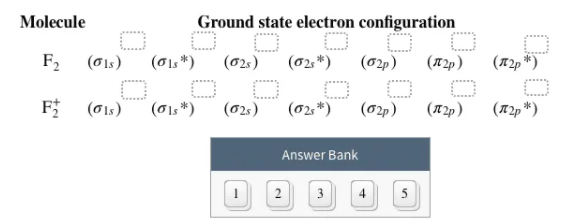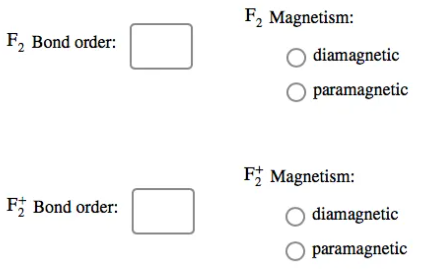# Problem: Use the molecular orbital theory to determine the ground state electron configuration of F2 and F2+.Then use the molecular orbital theory to determine the bond order and magnetism of the molecules.

###### FREE Expert Solution

We’re being asked to determine the ground state electron configuration of F2 and F2+ then determine the bond order and magnetism of the molecules

Recall that for:

• diamagnetic: all of the electrons are paired

• paramagnetic: at least one electron is unpaired

We can calculate the bond order using:

The bonding MOs are those without an asterisk (e.g., σ1s), while the antibonding MOs are those with an asterisk (e.g., σ1s*)

To do so, we need to look at the molecular orbital (MO) diagram of each molecule.

Recall that we have two types of MO diagrams:

81% (160 ratings)###### Problem Details

Use the molecular orbital theory to determine the ground state electron configuration of F2 and F2+.Then use the molecular orbital theory to determine the bond order and magnetism of the molecules.